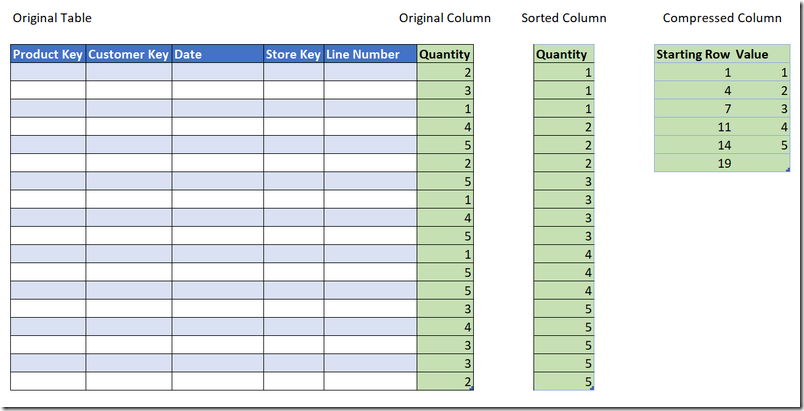Select PageLevel: Intermediate

There is a concept in the DAX language called “Syntax Sugar”. Simply put, the developers have created simplified versions of more complex formulas to make it easier for people to learn and use the DAX language. Here are a couple of examples

```Total Sales Syntax Sugar = SUM(Sales[ExtendedAmount])

Total Sales Full Syntax = SUMX(Sales,Sales[ExtendedAmount])

Total Sales Prior Year Syntax Sugar =
CALCULATE([Total Sales],SAMEPERIODLASTYEAR(Calendar[Date])

Total Sales Prior Year Full Syntax =

Simple Syntax in CALCULATE

Another example of syntax sugar is the simple syntax inside CALCULATE. There are 2 different ways you can apply a filter inside CALCULATE

```= CALCULATE(<expression>,Table[Column] compared to a scalar value,...)

= CALCULATE(<expression>,TableFunction(),...)```

Consider the following example using the simple syntax.

```Total Sales of Bikes Simple =
CALCULATE([Total Sales],Product[Category] = "Bikes")```

The above formula is syntax sugar for the following full formula.

```Total Sales of Bikes Full Version =
CALCULATE([Total Sales],
FILTER(ALL(Product[Category]),Product[Category] = "Bikes")
)```

As you can see, the simple version is clearly easier for a beginner to learn and understand. Under the hood, the simple version is converted to the full version prior to execution.

But Why use ALL(Table[Column])?

One thing a curious mind may be interested in is “why does the full version use ALL(Table[Column]) and not ALL(Table)”? Before I answer that question, let me first talk about compression. The Vertipaq engine (used in Power BI and Power Pivot) is a column store database. Traditional databases (eg SQL Server) use row store technology – each row is stored one full row at a time. A column store database stores the data one column at a time. Some of the consequences (benefits) of a column store database are that it is very efficient to filter, iterate and operate over entire columns of data in a table.

Compression Explained

There are a few different types of compression used in a the Vertipaq database, and I am only going to discuss one of them here. Let’s assume you have a data table with 6 columns and 100 million rows, and one of the columns is “Quantity”. The objective is to sum the quantity and come up with a total. A row store database (without an index) would need to retrieve all 100 million rows of data, including those columns not needed in the calculation, to come up with the answer. A column store database can directly access the single column to get the answer. What’s more, the columns can be compressed by the Vertipaq engine prior to being stored and accessed. One compression method is called run length encoding (RLE).

In the image below, the original column of data contains various values (in this case, the numbers 1 to 5). The way RLE works is that it first sorts the column, then creates a compressed version of the column, similar to that shown below (illustrative).As you can see above, the compressed version of the column is small compared to the original. In my example the original column/table has only 18 rows, but if the original table had 100 million rows with values from 1 to 5, it would still compress to exactly the same compressed version as shown above. The calculation to add up the columns in my sample above would therefore look something like this (pseudo code).

```Value 1 exists 3 times (starting row 4 minus 1).  Multiply 1 * 3

Value 2 exists 3 times (starting row 7 minus 4).  Multiply 2 * 3

Value 3 exists 4 times (starting row 11 minus 7).  Multiply 3 * 4

Value 4 exists 3 times (starting row 14 minus 11).  Multiply 4 * 3

Value 5 exists 5 times (starting row 19 minus 14).  Multiply 5 * 5

As you can no doubt understand, the above process is identical for an 18 row column and a 100 million row column. What’s more, the Vertipaq engine is mutli-threaded. If you have 4 cores on your machine, the calculations can be shared amongst the cores and completed in parallel before finally being combined to return the final result.

It follows that the more unique values in a column, the lower the compression. There also comes a tipping point where it is better just to store the uncompressed column than it is to try to compress the data. This is why it is better to remove un-needed precision and uniqueness from a column before loading. Examples include rounding to 2 decimal places rather than keeping unrounded decimal numbers, separating date and time into 2 columns and rounding the time to minutes rather than keeping the seconds (if seconds are not needed) etc.

Back to ALL(Table[Column])

Now, back to the syntax sugar. The reason it is more efficient to filter, iterate and operate over a column than a table is because the engine can iterate over the compressed version of the column. the ALL() function returns a table that contains all the distinct values in a column. This is exactly what the “Value” column in the compressed table in the image above contains (except the blank row at the bottom). So that is the reason the syntax sugar is written the way it is – it is to leverage the efficiencies of the Vertipaq engine.